RUSSIAN JOURNAL OF EARTH SCIENCES, VOL. 20, ES5002, doi:10.2205/2020ES000701, 2020

# Assessment the effect of atmospheric correction algorithms for monitoring PM10 concentration by using Landsat 8OLI data: A case study in Hanoi, Vietnam

Nguyen Nhu Hung1, Le Minh Hang1, Tran Van Anh2, Le Vu Hong Hai1

1Le Quy Don Technical University, Hanoi, Vietnam

2Faculty of Geomatics and Land Administration, University of Mining and Geology, Hanoi, Vietnam

### Abstract

Air pollution is becoming more serious, especially in developing countries like Vietnam. Air pollution is affecting human health, especially atmospheric particulate matter (PM) with a diameter 2.5 $\mu$m (PM2.5) or 10 $\mu$m (PM10). Atmospheric particulate matter affect to scatter or absorb electromagnetic energy which reflect to satellite sensors. Hence, PM10 concentration can be determined based on ground measured data or satellite images. PM10 concentration is determined by multiple linear regression method using field measured value and atmospheric reflectance calculated by satellite images at the same time. The atmospheric reflectance is defined as the subtraction of top of atmospheric reflectance (TOA) and surface reflectance value. Therefore, atmospheric correction algorithm for surface reflectance plays an important role in determining PM10 concentration by using remote sensing data. In this article, the authors presented the assessment effect of atmospheric correction methods as DOS (Dark Object Subtraction), FLAASH (Fast Line-of-sight Atmospheric Analysis of Hyper cubes) and LaSRC (Landsat 8 Surface Reflectance Code) for accurate regression of PM10 concentration from satellite images. The experimental data were Landsat 8OLI of Hanoi area, Vietnam at three times on 22 January 2015; 30 May 2015 and 12 October 2016.

### 1. Introduction

Air pollution is seriously affecting human health in many countries in the world, especially in developing countries like Vietnam. Private vehicles and industries have dramatically increased; consequently, air pollution becomes more serious. Viet Nam is one of ten countries with the worst air pollution among 132 countries whose environments were surveyed with effects on human health based on the 2012 Environmental Performance Index released at World Economic Forum in Davos. Air pollution is mostly concentrated in urban, industrial parks and construction areas. Atmospheric aerosol particles which are known as particulate matter (PM) are microscopic solid or liquid matter suspended in the atmosphere of Earth. PM10 are coarse particles with a diameter 10 micrometers ($\mu$m). Medical experts said that small particles like PM2.5 or PM10 could seriously affect human health which cause the risk for respiratory and lung diseases.

Many studies have shown that satellite imagery can identify air pollution, especially PM2.5 or PM10. The method of determining PM2.5 or PM10 concentration from satellite images is usually based on the correlation regression between ground measurement data and aerosol optical thickness values determined from satellite images at the same time [Forster, 1984; Jerome, 2010; Hadjimitsis,1999; Hadjimitsis et al., 2003, 2004, 2010]. In these methodologies, aerosol optical thickness, which is the most important unknown of the atmospheric correction algorithm to solve the equation for radiation transmission and removal of atmospheric effects from remote satellite imagery, is the main parameter to determine PM10 [Hadjimitsis,1999; Hadjimitsis et al., 2002, 2004; 2007; Forster, 1984]. The aerosol optical thickness has been used as a tool for assessing air pollution using satellite images [Kanaroglou et al., 2002; Kaufman et al., 1990]. Nadzri et al.  proved aerosol optical thickness as a linear function of atmospheric reflectance, which corresponds to each optical satellite image. As a result, atmospheric reflectance is a linear function with PM10 concentration. The atmospheric reflection is defined as the subtraction of top of atmosphere reflectance and surface reflectance value. Therefore, the determination of air pollution through the derivation of the atmospheric reflectance from the satellite images can be assessed the atmospheric correction algorithms.

Satellite images data in these studies were moderate resolution as MODIS, LANDSAT [Dinoi et al., 2010; Gao et al., 2010; Nordio et al., 2013; Zhang et al., 2015]) and high resolution as SPOT [Sifakis et al., 1992]. However, DOS (Dark Object Subtraction) algorithm is usually applied for correcting the effect of atmospheric in satellite images in determining the absorption and scattering of electromagnetic energy to reach at satellite sensors [Hadjimitsis,2009; Kyriacos, 2011]. In addition, atmospheric correction algorithms of ATCOR software [Lim et al., 2007] or surface reflectance data from USGS [Roy et al., 2017] were used to determine surface reflectance value of satellite images. DOS is an atmospheric correction algorithm assumes that there are features which have near-zero percent reflectance (i.e., water, shadow...) by additive scattering from remote sensing data. FLAASH (Fast Line-of-sight Atmospheric Analysis of Hypercubes) is built into a commercial image processing software as ENVI and corrects the effect of the atmosphere in satellite image by the hypothetical atmospheric model. LaSRC (Landsat 8 Surface Reflectance Code) is an atmospheric correction algorithm for only Landsat 8 by USGS's and uses the atmospheric information at an acquired time. However, few studies assess the effect of atmospheric correction methods for accuracy and correlation coefficient in determining PM10 concentration from satellite images. Therefore, in this article, the authors present the experience results and evaluate the effect of atmospheric correction algorithms as DOS, FLAASH, and LaSCR on the accuracy of determining PM10 concentration by satellite images.

### 2. Study Area and Materials

#### 2.1. Study Area

Hanoi is located between $20\mbox{°} 53'$ to $21\mbox{°} 23'$ North latitude and $105\mbox{°} 44'$ to $106\mbox{°} 02'$ East longitude.The Red River is the main river in Hanoi. The Red River flows through Hanoi from Dong Anh district to Thanh Tri district is 40 km long. Besides, Hanoi has many waterways, though smaller and shorter, and lakes are connecting with the main rivers of Hanoi. The climate of Hanoi is a warm humid subtropical climate. Hanoi has the typical climate of Northern Vietnam, with four distinct seasons. Summer, from May until August, is characterized by hot and humid weather and rainy. September and October comprise the fall season, characterized by a decrease in temperature. Winter, from November to January, is dry and cool by national standards. Hanoi is usually cloudy and foggy in winter. Spring, from February to April, is humid weather and drizzly.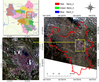Figure 1

Hanoi has a dramatic urbanization rate of 69.7% and is the second city of Vietnam after Ho Chi Minh City. As a result, air pollution is becoming more serious and a challenge for Hanoi. The air pollution in Hanoi is mainly concentrated in center districts of Hanoi. Hence, the study area is chosen in the central area with the districts of Dong Da, Hoan Kiem, Ba Dinh, Hai Ba Trung, Cau Giay, Thanh Xuan and Tay Ho (Figure 1).

#### 2.2. Experience Materials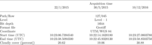Table 1

Landsat 8OLI data is used for determining PM10 concentration in our study. Landsat 8 images were acquired at the time of synchronization with PM10 collected in the field. There were three Landsat 8OLI scenes which corresponded to the typical weather conditions that are distinctive for each of the seasons in Vietnam, such as 22 January 2015; 30 May 2015; 10 December 2016. The characteristics of the experience materials are shown in Table 1.

#### 2.3. Field Surveying Data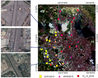Figure 2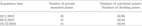Table 2

The field collecting PM10 data was measured simultaneously with Landsat 8 satellite images acquisition (see Figure 2 and Table 2). The Dust Trak II instrument was used to collect field data. Each sample point was positioned by the GPS device and recorded the measurement time. Figure 2a, Figure 2b, Figure 2c were seven checking points which were the same locations in three times of image acquisitions.

### 3. Methodology

The method of PM10 estimation proposed in this study included four main steps such as: (i) Pre-processing Landsat data (convert digital values to TOA and surface reflectance); (ii) Calculating atmospheric reflectance (subtraction of TOA and surface reflectance of checking points); (iii) Multiple linear regression PM10 concentration based on atmospheric reflectance and ground measurement data; (iv) Validation of results. In this article, we experienced three atmospheric corrections algorithm as DOS, FLAASH, and LaSRC for estimating PM10 concentration by Landsat 8OLI and surveying data.

#### 3.1. Pre-Processing Landsat 8OLI Data

The pre-processing image is carried out by transforming digital values (DN) into surface reflectance. There are several steps to calibrate the radiation, in which: convert the DN into the radiation value on the top of the atmosphere reflectance (TOA), then transform of TOA spectral reflectance to surface reflectance.

Landsat 8OLI data can also be converted to TOA reflectance using reflectance rescaling coefficients provided in the product metadata file (MTL file). The following (1) is used to convert DN values to TOA reflectance for OLI data as follows (Landsat, (n.d.), https://www.usgs.gov/land-resources/nli/landsat/using-usgs-landsat-level-1-da ta-product:

\begin{equation} \tag*{(1)} \rho_{\lambda '}=M_{\rho} \times Q_{\mathrm{cal}}+A_{\rho} \end{equation}

where: $\rho_{\lambda}'$: TOA planetary reflectance, without correction for solar angle. Note that $\rho_{\lambda}'$ does not contain a correction for the sun angle; $M_{\rho}$: Band-specific multiplicative rescaling factor from the metadata (REFLECTANCE_MULT_BAND$_x$, where $x$ is the band number). $A_{\rho}$: Band-specific additive rescaling factor from the metadata (REFLECTANCE_ADD_BAND$_x$, where $x$ is the band number) $Q_{\mathrm{cal}}$: Quantized and calibrated standard product pixel values (DN).

TOA reflectance with a correction for the sun angle is then (Landsat, (n.d.), https://www.usgs.gov/ land-resources/nli/landsat/using-usgs-landsat-level-1-data-product:

\begin{eqnarray*} \rho {\lambda }=\frac{\rho \lambda'}{\cos(\theta_{SZ})} = \frac{\rho \lambda'}{\sin(\theta_{SE})} \end{eqnarray*}

where: $\rho_{\lambda}$ – TOA planetary reflectance; $\theta_{SE}$ – local sun elevation angle. The scene center sun elevation angle in degrees is provided in the metadata (SUN_ELEVATION). $\theta_{SZ}$ – local solar zenith angle; $\theta_{SZ}= 90\mbox{°} -\theta_{SE}$.

Dark Object Subtraction (DOS) method was proposed by Chavez  and determined the path radiation in atmospheric of electromagnetic waves [Sobrino et al., 2004]. With the DOS method depends on the definition of $T_V$ parameters, $T_Z$ and $E_{\mathrm{down}}$ that divided into different methods (DOS1, DOS2, DOS3, DOS4) have different precision. In this study, we used DOS1, where the parameters are determined according to: $T_V=1$; $T_Z=1$; $E_{\mathrm{down}} =0$ [Moran et al., 1992]. For Landsat 8 OLI, surface reflectance is calculated according to as the formula following:

\begin{eqnarray*} \rho =\frac{\pi(\rho_\lambda - L_P) \; d^2} {\mathrm{ESUN}_{\lambda} \; \cos(\theta_{SZ})} \end{eqnarray*}

where: $L_P$ – path radiance;

ESUN$_\lambda$ – mean solar exo-atmospheric irradiances; $d$ – earth–sun distance (provided in the Landsat 8 metadata file).

FLAASH (Fast Line-of-sight Atmospheric Analysis of Hypercubes) used a standard equation for spectral radiance at a sensor pixel as follows:

\begin{eqnarray*} L=\frac{A \rho }{1- \rho_e S} + \frac{B \rho_e }{1- \rho_e S} + L_a \end{eqnarray*}

where: $\rho$ – the pixel surface reflectance; $\rho_e$ – an average surface reflectance for the pixel; a surrounding region $S$ – the spherical albedo of the atmosphere; $L_a$ – the radiance back scattered by the atmosphere; $A$ and $B$ are coefficients that depend on atmospheric and geometric conditions.

The values of $A$, $B$, $S$ and $L_a$ parameters were calculated by using MODTRAN4 that are relative with the viewing angles, solar angles and the mean surface elevation of the measurement. MOTRAN4 assumes a certain model atmosphere, aerosol type, and visible range. The solution method involves computing a spatially averaged radiance image $L_a$, from which the spatially averaged reflectance $\rho_e$ is estimated using the approximate equation:

\begin{eqnarray*} L_e\approx \frac{(A + B) \rho_e }{1- \rho_e S} + L_a \end{eqnarray*}

LaSRC (Landsat 8 Surface Reflectance Code) which is a surface reflectance algorithm for only Landsat 8, is used by USGS (Landsat, n.d.). MOFIS CMA determines water vapour and air temperature. The surface pressure is calculated internally based on the elevation. The radiative transfer model is an internal algorithm which is built by USGS.

#### 3.2. Calculating Atmospheric Reflectance and Multiple Linear Regression PM10 Concentration

Solar energy enters the aerosol of the troposphere, under the impact of pollutant gas molecules and dust particles, partially reflected in the aerosol layer and then toward the satellite's receiver, the sun's rays reach the objects on the surface and then reflect to the satellite sensor. Based on the energy loss to the satellite receiver due to absorption, scattering of pollutant gases and dust particles from which the dust content in the air is calculated. As a result, AOT or atmospheric reflectance can be calculated by subtraction of TOA value and surface reflectance of Landsat 8 OLI data.

The relationship between PM concentration and atmospheric reflectance originates from a homogeneous atmosphere that contains globular spheres. Concentration at the surface is obtained after drying of the air sample given by [Koelemeijer et al., 2006]. Therefore, PM10 concentration parameters can correlate better with direct atmospheric reflectance and calculated by (2) [Lim et al., 2004; Nadzri et al., 2010]

\begin{equation} \tag*{(2)} \mathrm{PM10} = a_0 R_{\lambda 1} + a_jR_{\lambda 2} + a_2R_{\lambda 3} + \ldots \end{equation}

where: $R_{\lambda i}$ is the atmospheric reflectance ($i = 1$, 2 and 3 corresponding to wavelength for satellite); $a_j$ is the algorithm coefficient ($j = 0$, 1 and 2) obtained from experiment.

### 4. Results and Discussion

#### 4.1. Assessment Atmospheric Correction Algorithms for Determing PM10 Concentration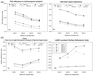Figure 3

To assess the effect of atmospheric correction algorithms on atmospheric reflectance values based on the characteristic of spectral reflectance at 03 checking points of the same object at different times, Figure 3 shows the spectral graphs of three atmospheric correction methods at 03 checking points.

The locations of the checking points in Figure 3 are in Pham Van Dong street, Hanoi, Vietnam (Figure 2a, Figure 2b, Figure 2c). Hence, these points have the same characteristics of spectral reflectance. In Figure 3, the surface reflectance values were determined by using different atmospheric correction methods. Figure 3a shows TOA reflectance values. The same positions have similar characteristics of TOA reflectance. Figure 3b shows the surface reflectance line by using the DOS method at ground measured points. DOS1 method used a constant parameter so that the surface reflectance line of DOS is similar to TOA reflectance line. Figure 3c, Figure 3d show the surface reflectance values which are determined by the LaSRC method and the FLAASH method. FLAASH method, which is an atmospheric method, uses a hypothetical model. The checkpoints' surface reflectance line on 22 January 2015, 30 May 2015, and 10 December 2016 have the same value. However, the surface reflectance values in checkpoints at multi-temporal, corrected by the DOS method and the FLAASH method are differences (Figure 3b, Figure 2c). According to that, the influence of the atmospheric uncorrected absolutely by DOS and Flaash method because of using a hypothetical atmospheric model.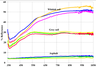Figure 4

LaSRC is a method that applies for to 8 data and uses meteorological conditions at the time of image acquisition. The surface reflectance values of points 2.1 and 2.2 on 30 May 2015 and points 3.1 and 3.2 on 10 December 2016 are relatively similar. The surface reflectance of points 1.1 and 1.2 on 22 January 2015, which affected by cloudy and haze, had not been eliminated by atmospheric correction. As a result that the surface reflectance values of checking point at the same objects are similar value in multi-temporal images. The comparisons between the spectral reflectance of Point 2 and Point 3 in Figure 3d and the spectral reflectance of Asphalt in Figure 4 are the same. Therefore, the LaSRC algorithm is suitable for correcting the effective atmospheric and pre-processing images of multi-temporal Landsat 8OLI images.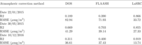Table 3

The effect of atmospheric correction method's effects of determining PM10 concentration based on satellite images and ground measured points were approved by the multiple regression ANOVA. In this study, PM10 concentration was regressed by using atmospheric reflectance calculated by four bands of Landsat 8OLI (Band 1, Band 2, Band 3, Band 4) and ground measured points at the same time. The accuracy assessment includes in correlation coefficient ($R^2$), and root mean square error (RMSE) of multiple linear regression by ANOVA (Analysis of Variance) method. Table 3 shows the analysis of PM10 multiple regression using different atmospheric correction methods by ANOVA.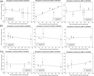Figure 5

Figure 5 shows the correlation of linear regression and residuals between PM10 concentration by ground measurement and PM10 concentration calculated from ANOVA regression results in Table 3 of the checkpoints on 22 January 2015 and 30 May 2015. The standard errors of determining PM10 concentration from Landsat 8OLI images data corrected by LaSRC algorithm were lower than the others.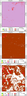Figure 6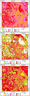Figure 7

According to the results in Table 3 and Figure 6 and Figure 7, it proved that LaSRC had corrected the effect of atmospheric in satellite images better than DOS and FLAASH method which the RMSEs were 33.2 $\mu$g/m$^3$ (22 January 2015), 34.4 $\mu$g/m$^3$ (30 May 2015) and 13.74 Day/m$^3$ (10 December 2016) and $R$-square were 0.996 (22 January 2015), 0.987 (30 May 2015) and 0.919 (10 December 2016).

### 5. Conclusion

In conclusion, PM10 concentration was determined by multiple linear regression methods by AOT value determined from Landsat 8OLI image and ground measured data. In this study, AOT values were calculated by TOA value and surface reflectance value of Landsat 8 images. The result of the atmospheric correction of Landsat 8 images is the surface reflectance image. The results of our experiment are proved the effects of atmospheric correction methods on the accuracy and correlation coefficient of multiple regression PM10 concentration by AOT values and field surveying values. LaSRC atmospheric calibration method built for only Landsat 8 of USGS had higher accuracy and correlation coefficient than DOS, FLAASH methods (accuracy archived 33.15 and $R^2$ was 0.98). Besides, the LaSRC method corrected the atmospheric effects better than the others on multi-temporal satellite images. The surface reflectance values determined by the atmosphere correction method at the same object at different times had the same value. It is a requirement for the analysis of multi-temporal satellite images. According to the results of the assessment, the effects of atmospheric correction for determining PM10 concentration by using remote sensing data, we choose LaSRC which was an appropriate atmospheric correction method to determine AOT value and build the correlation function between AOT value and ground measured data simultaneously.

### References

Chavez, P. S. (1996) , Image-based atmospheric corrections-revisited and improved, Photogrammetric Engineering and Remote Sensing, 62, no. 9, p. 1025–1035.

Dinoi, A., M. A. Perrone, P. Burlizzi (2010) , Application of MODIS Products for Air Quality Studies OverSoutheastern Italy, Remote Sensing, 2, p. 1767–1796, https://doi.org/10.3390/rs2071767.

Forster, B. C. (1984) , Derivation of atmospheric correction procedures for Landsat MSS with particular reference to urban data, International Journal of Remote Sensing, 5, p. 799–817.

Gao, J., Y. Zha (2010) , Meteorological Influence on Predicting Air Pollution from MODIS-Derived Aerosol Optical Thickness: A Case Study in Nanjing, China, Remote Sensing, 2, p. 2136–2147, https://doi.org/10.3390/rs2092136.

Hadjimitsis, D. G. (1999) , The application of atmospheric correction algorithms in the satellite remote sensing of reserviors, PhD thesis, Univeristy of Surrey, School of Engineering in the Environment Department of Civil Engineering Guidford, Surrey.

Hadjimitsis, D. G. (2009) , Aerosol optical thickness (AOT) retrieval over land using satellite image-based algorithm, Air Quality, Atmosphere &amp; Health, 2, p. 89–97, https://doi.org/10.1007/s11869-009-0036-0.

Hadjimitsis, D. G., C. R. I. Clayton, V. S. Hope (2004) , An assessment of the effectiveness of atmospheric correction algorithms through the remote sensing of some reservoirs, International Journal of Remote Sensing, 25, no. 18, p. 3651–3674, https://doi.org/10.1080/01431160310001647993.

Hadjimitsis, D. G., C. R. I. Clayton, A. Retalis (2003) , Darkest pixel atmospheric correction algorithm: a revised procedure for environmental applications of satellite remotely sensed imagery, Proceedings of the 10th International Symposium on Remote Sensing, organised by NASA, SPIE Conference, 414, p. 8–12, NASA, Barcelona, Spain.

Hadjimitsis, D. G., P. T. Kyriacos, P. Giorgos (2008) , The application of atmospheric correction algorithms for monitoring atmospheric pollution using Landsat TM images, Proc. SPIE 7107, Remote Sensing of Clouds and the Atmosphere XIII, 71070H (13 October 2008), SPIE, Cardiff, Wales, United Kingdom, https://doi.org/10.1117/12.800346.

Hadjimitsis, D. G., A. Retalis, C. R. I. Clayton (2002) , The assessment of atmospheric pollution using satellite remote sensing techniques in large cities in the vicinity of airports, Water, Air and Soil Pollution: Focus. An International Journal of Environmental Pollution, 2, no. 5–6, p. 631–640, https://doi.org/10.1023/A:1021305417004.

Hadjimitsis, D. G., A. Nisantzi, K. Themistocleous (2010) , Satellite remote sensing, GIS and sun-photometers for monitoring PM10 in Cyprus, Proc. of SPIE, 7826, https://doi.org/10.1117/12.865120.

Jerome, V., S. Richard, R. Didier (2010) , Atmospheric particulate matter (PM) estimation from SeaWiFS imagery, Remote Sensing of Environment, p. 1–10, https://doi.org/10.1016/j.rse.2007.03.009.

Kanaroglou, P. S., N. A. Soulakellis, N. I. Sifakis (2002) , Improvement of satellite derived pollution maps with the use of a geostatistical interpolation method, Journal of Geographical Systems, 4, p. 193–208, https://doi.org/10.1007/s101090100080.

Kaufman, Y. J., R. S. Fraser, R. A. Ferrare (1990) , Satellite remote sensing of large scale air pollution method, Journal of Geophysical Research, 95, p. 9895–9909, https://doi.org/10.1029/JD095iD07p09895.

Koelemeijer, R. B. A., C. D. Homan, J. Matthijsen (2006) , Comparison of spatial and temporal variations of aerosol opticalthickness and particulate matter over Europe, Atmospheric Environment, 40, p. 5304–5315, https://doi.org/10.1016/j.atmosenv.2006.04.044.

Kyriacos, T. (2011) , Improving atmospheric correction methods for aerosol optical thickness retrieval supported by in-situ observations and GIS analysis, Doctor of Philosophy Dissertation, Cyprus University of Technology, Limassol.

Lim, H. S., M. Z. MatJafri, K. Abdulla (2004) , Remote Sensing of PM10 From Landsat TM Imagery, 25th ACRS 2004 Chiang Mai, p. 739–744, Chiang Mai, Thailand.

Lim, H. S., Z. M. J. Mohd (2007) , Mapping atmospheric pollution using remote sensing, SPIE Newsroom, https://doi.org/10.1117/2.1200710.0853.

Moran, M. S., D. J. Ray, N. S. Philip, et at. (1992) , Evaluation of simplified procedures for retrieval of land surface reflectance factors from satellite sensor output, Remote Sensing of Environment, 41, no. 2–3, p. 169–184, https://doi.org/10.1016/0034-4257(92)90076-V.

Nadzri, O., Z. M. J. Mohd, H. S. Lim (2010) , Estimating Particulate MatterConcentration over Arid Region Using Satellite Remote Sensing: A CaseStudy in Makkah, Saudi Arabia, Modern Applied Science, 4, p. 131–142, https://doi.org/10.5539/mas.v4n11p131.

Nordio, F., I. Kloog, B. Coull, et at. (2013) , Estimating spatio-temporal resolved PM10 aerosol massconcentrations using MODIS satellite data and land use regression over Lombardy, Italy, Atmospheric Environment, 74, p. 227–236, https://doi.org/10.1016/j.atmosenv.2013.03.043.

Roy, A., A. Jivani, B. Parekh (2017) , Estimation of PM10 Distribution using Landsat 7 ETM+ Remote Sensing Data, International Journal of Advanced Remote Sensing and GIS, 6, no. 1, p. 2246–2252, https://doi.org/10.23953/cloud.ijarsg.284.

Sifakis, N., P. Y. Deschamps (1992) , Mapping of Air Pollution Using SPOT Satellite, Photogrammetric Engineering &amp; Remote Sensing, 5, p. 4.

Sobrino, J. A., C. J-M. Juan, L. Paolini (2004) , Land surface temperature retrieval from Landsat TM 5, Remote Sensing of Environment, 90, p. 434–440, https://doi.org/10.1016/j.rse.2004.02.003.

Zhang, L., et al. (2015) , Retrieval of aerosol optical depth over Beijing using Landsat8/OLI data, Proc. SPIE 9610, Remote Sensing and Modeling of Ecosystems for Sustainability XII, p. 961,011, SPIE Optical Engineering + Applications, San Diego, California, https://doi.org/10.1117/12.2186761.

Received 7 October 2019; accepted 18 December 2019; published 17 August 2020.Citation: Hung Nguyen Nhu, Le Minh Hang, Tran Van Anh, Le Vu Hong Hai (2020), Assessment the effect of atmospheric correction algorithms for monitoring PM10 concentration by using Landsat 8OLI data: A case study in Hanoi, Vietnam, Russ. J. Earth Sci., 20, ES5002, doi:10.2205/2020ES000701.

Generated from LaTeX source by ELXfinal, v.2.0 software package.